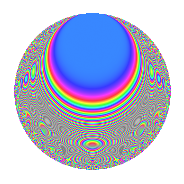# Properties

 Label 187.2.hLevel 187 Weight 2 Character orbit h Rep. character $$\chi_{187}(100,\cdot)$$ Character field $$\Q(\zeta_{8})$$ Dimension 56 Newforms 1 Sturm bound 36 Trace bound 0

# Related objects

## Defining parameters

 Level: $$N$$ = $$187 = 11 \cdot 17$$ Weight: $$k$$ = $$2$$ Character orbit: $$[\chi]$$ = 187.h (of order $$8$$ and degree $$4$$) Character conductor: $$\operatorname{cond}(\chi)$$ = $$17$$ Character field: $$\Q(\zeta_{8})$$ Newforms: $$1$$ Sturm bound: $$36$$ Trace bound: $$0$$

## Dimensions

The following table gives the dimensions of various subspaces of $$M_{2}(187, [\chi])$$.

Total New Old
Modular forms 80 56 24
Cusp forms 64 56 8
Eisenstein series 16 0 16

## Trace form

 $$56q - 16q^{6} + O(q^{10})$$ $$56q - 16q^{6} - 16q^{10} - 16q^{14} + 24q^{15} - 32q^{16} + 8q^{17} - 24q^{19} + 16q^{20} - 24q^{24} - 8q^{25} - 48q^{27} - 40q^{32} + 16q^{33} + 64q^{34} + 32q^{35} + 64q^{36} + 8q^{37} - 32q^{39} + 96q^{40} - 24q^{41} - 8q^{42} - 32q^{43} + 16q^{44} - 32q^{45} - 16q^{46} - 24q^{48} - 112q^{50} - 48q^{51} + 8q^{53} - 72q^{54} + 64q^{56} + 40q^{57} + 16q^{58} + 16q^{59} - 8q^{60} - 64q^{61} + 56q^{62} + 16q^{63} + 56q^{65} + 24q^{67} - 88q^{68} - 64q^{69} - 96q^{70} - 16q^{71} + 8q^{73} - 48q^{74} + 40q^{75} + 88q^{76} + 136q^{78} - 32q^{80} + 104q^{82} - 56q^{83} + 80q^{84} - 8q^{85} - 32q^{86} + 56q^{87} - 32q^{91} + 40q^{92} + 8q^{93} + 16q^{94} + 48q^{95} + 64q^{96} - 16q^{97} + O(q^{100})$$

## Decomposition of $$S_{2}^{\mathrm{new}}(187, [\chi])$$ into irreducible Hecke orbits

Label Dim. $$A$$ Field CM Traces $q$-expansion
$$a_2$$ $$a_3$$ $$a_5$$ $$a_7$$
187.2.h.a $$56$$ $$1.493$$ None $$0$$ $$0$$ $$0$$ $$0$$

## Decomposition of $$S_{2}^{\mathrm{old}}(187, [\chi])$$ into lower level spaces

$$S_{2}^{\mathrm{old}}(187, [\chi]) \cong$$ $$S_{2}^{\mathrm{new}}(17, [\chi])$$$$^{\oplus 2}$$